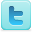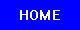﻿ Trajectory & Range of a Projectile Experiments and Background Information
Trajectory & Range of a Projectile
K-12 Experiments and Background Information

Projectile Experiments

Projectile Background Information

Definitions

The ballistic trajectory of a projectile is the path that a thrown object will take under the action of gravity, neglecting all other forces, such as friction from air resistance, or propulsion.

In physics, a projectile launched with specific initial conditions in a uniform gravity field will have a predictable range.

Topics of Interest

This article provides a list of methods for calculating the trajectory and range of a projectile under the influence of Earth's gravity.

In the equations on this page, the following variables will be used:

• g: the gravitational acceleration—usually taken to be 9.81 m/s2 near the Earth's surface
• θ: the angle at which the projectile is launched
• v: the velocity at which the projectile is launched
• y0: the initial height of the projectile
• d: the total horizontal distance traveled by the projectile

## Conditions at the final position of the projectile

### Distance traveled

The total horizontal distance (d) traveled.When the surface the object is launched from and is flying over is flat, the distance traveled is: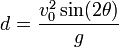As a special case, the distance is given by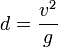when the angle (θ) is 45° and the initial height (y0) is 0.

### Time of flight

The time of flight (t) is the time it takes for the projectile to finish its trajectory.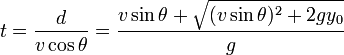As above, this expression can be reduced to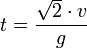if θ is 45° and y0 is 0.

### Angle of reach

The "angle of reach" (not quite a scientific term) is the angle (θ) at which a projectile must be launched in order to go a distance d, given the initial velocity v.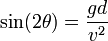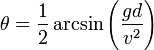Range of a Projectile

In physics, a projectile launched with specific initial conditions in a uniform gravity field will have a predictable range. As in Trajectory of a projectile, we will use:

• g: the gravitational acceleration—usually taken to be 9.81 m/s2 near the Earth's surface
• θ: the angle at which the projectile is launched
• v: the velocity at which the projectile is launched
• y0: the initial height of the projectile
• d: the total horizontal distance travelled by the projectile

When neglecting air resistance, the range of a projectile will beIf (y0) is taken to be zero, meaning the object is being launched on flat ground, the range of the projectile will then simplify to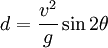For more calculations:
https://en.wikipedia.org/wiki/Range_of_a_projectile
https://en.wikipedia.org/wiki/Trajectory

Source: Wikipedia (All text is available under the terms of the GNU Free Documentation License and Creative Commons Attribution-ShareAlike License.)

Science Fair Projects Resources
Engineering Science Fair Books